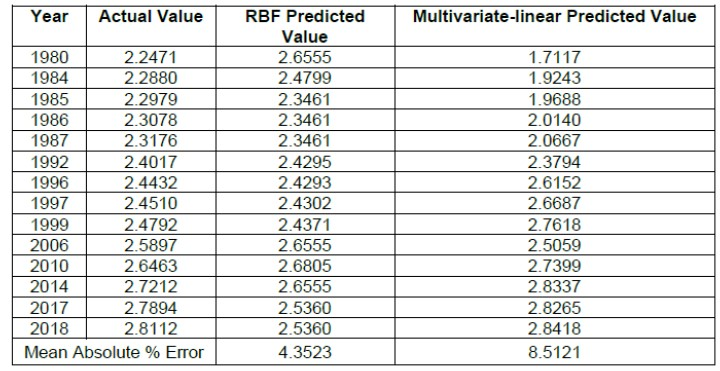Wu, X., Bao, Q., and Liu, G. (2022). "Comparative analysis of dynamic changes in forest resources with RBF neural network and regression method," BioResources 17(2), 2313-2330.

#### Abstract

Forest resources are the most important natural resources; their dynamic changes (growth or decline) are affected by socio-economic factors, and to study their linkage is of great significance. However, the relationship between forest resources and social economic factors is normally a multivariate nonlinear relationship. There are difficulties in accurately analyzing it by using traditional multivariate-statistical methods. Also, its explicit mathematical model is inconvenient for intelligent management. In this paper, the radial basis function (RBF) neural network was introduced to study the relationship between the changes of forest resources and socio-economic factors and was evaluated by comparison with the traditional multiple-linear regression model. The results showed that the RBF neural network method can be applied in modeling the dynamic changes of forest resources and showed a higher prediction accuracy over the traditional statistical modeling approaches. At the same time, the RBF neural network can analyze and evaluate the importance of influencing factors simply and conveniently. The results provide a new way and show an application potential for the analysis and intelligent management in forest resources.

Comparative Analysis of Dynamic Changes in Forest Resources with RBF Neural Network and Regression Method

Xiaoyu Wu, Qingfeng Bao,* and Guiyan Liu

Forest resources are the most important natural resources; their dynamic changes (growth or decline) are affected by socio-economic factors, and to study their linkage is of great significance. However, the relationship between forest resources and social economic factors is normally a multivariate nonlinear relationship. There are difficulties in accurately analyzing it by using traditional multivariate-statistical methods. Also, its explicit mathematical model is inconvenient for intelligent management. In this paper, the radial basis function (RBF) neural network was introduced to study the relationship between the changes of forest resources and socio-economic factors and was evaluated by comparison with the traditional multiple-linear regression model. The results showed that the RBF neural network method can be applied in modeling the dynamic changes of forest resources and showed a higher prediction accuracy over the traditional statistical modeling approaches. At the same time, the RBF neural network can analyze and evaluate the importance of influencing factors simply and conveniently. The results provide a new way and show an application potential for the analysis and intelligent management in forest resources.

DOI: 10.15376/biores.17.2.2313-2330

Keywords: RBF neural network; Forest resources; Socio-economic factors; Nonlinear fitting; Intelligent management

Contact information: School of Economic Management, Inner Mongolia Agricultural University, Hohhot 010018, P.R. China; *Corresponding author: baoqingfengIMAU@163.com

INTRODUCTION

Forest resources are the most precious natural resources on the earth, which not only have important economic value, but also play an important role in protecting the biodiversity, improving the ecological environment, and mitigating the climate change (Javadinejad et al. 2021; Talebmorad et al. 2021). Therefore, to study the dynamic changes in forest resources is of great significance for the protection and the rational use of natural resources, and for the realization of the sustainable development in ecological environment and social economy (Zelazowski et al. 2011; Gandiaga and Moreau 2019). Forest resources can generally be measured by indicators such as forest coverage, tree stocking, forest area, etc., which reflects the forest in both “quantity” and “quality”. The factors affecting the growth and decline of forest resources are not only those of natural conditions (such as rainfall, soil quality, land surface temperature, etc.), but also socio-economic factors, including economic growth, population, industry structure, the application of science and technology, as well as the policy systems (such as deforestation, forest protection, and afforestation policies), etc. (Zhang et al. 2006; Ashraf et al. 2017; Okumua and Muchhapondwa 2020; Zhang and Ke 2020).

For a specific area, the natural condition, as an objective factor, has a relatively fixed impact on forest resources, while the socio-economic factors are varied and controllable, which has a special meaning to the dynamic changes in forest resources (Silva et al. 2016). This has attracted many scholars to study the effect of socio-economic factors on forest resources for coordinating their relationship and realizing the sustainable development. A general consensus is that economic growth has the greatest effect on the changes of forest resources. The earlier research studies applied a simple multiple-linear regression method with cross-sectional data to model the relationship between deforestation and economics (Allen and Barnes 1985; Kahn and McDonald 1995; Tole 1998). Later the panel data often have been used to overcome the limitations associated with cross-sectional data regression (Bhattarai and Hamming 2004; Ostad-Ali-Askari and Shayannejad 2021). Among the methods in studying the relationship between forest resources and economic growth, the more common and accepted method by most scholars is to use the environmental Kuznets curve (EKC) model (Ahmed et al. 2015; Waluyo and Terawaki 2016; Murshed et al. 2020). In recent years, researchers have considered more socio-economic factors and established the extended EKC models by adding some other impacting factors as the control variables, such as social economics, geographical features, policy factors, etc. (Culas 2007; Hao et al. 2019). Based on the EKC theory, most studies have set the regression model in quadratic-curve form and asserted that there was a “U-shaped” relationship between forest resources and economic benefits. That is, initially the economic development may bring damage to forest resources, but with the further economic development it may drive growth in forest resources (Caravaggio 2020). In this regard, there are also some disputes among academics, and different conclusions have been drawn. For example, some scholars believed that the EKC curve only exists in some regions, such as Latin America, but does not exist in other regions such as Africa (Koop and Tole 1999; Barbier and Burgess 2001). Some scholars even demonstrated that the relationship between forest resources and economic development is in the form of a cubic curve with an “N” shape. An example is provided in western China (Chen and Zhu 2020).

Although the traditional statistical techniques or mathematical models have the merits with convenient and fast in model setting, the following drawbacks exist: 1) The method needs to satisfy a linear relationship between independent and dependent variables, and the observations are independent of each other. There is no multicollinearity problem between the independent variables. In other words, there is no problem being unable to deal with the correlation and coupling problems between independent variables, and it is a linear model. In practice, the forest resources tend to be a vast distribution with abundant tree species, and the forest coverage area spans a wide range with large temporal and spatial differences. The dynamic change process of forest resources is essentially a nonlinear mapping process. In other words, the indicators of forest resources and their influencing factors are often non-linear, and the factors are not completely independent but coupled with each other. Therefore, the usage of traditional statistical methods to quantitatively solve the complex non-linear relationships typically results in a low precision problem; 2) Normally every explained variable (or dependent variable) for forest resources needs a multiple-linear regression model, and the complex explicit mathematical equations are not conducive to the realization of intelligent management in forest resources, especially in this big-data era (Stern et al. 1996; Pirnazar et al. 2018; Zambrano-Monserrate et al. 2018); and 3) The empirical analysis of dynamic changes in forest resources and their influential factors often include some qualitative components, which are hard to be integrated into mathematical equations, or some missing data is encountered, which creates lowered modeling accuracy.

To this end, this paper applied a radial basis function (RBF) neural network method to the study of forest resources. The RBF neural network presents a unique advantage in modeling nonlinear problems, and it has the characteristics of fault tolerance, self-learning, and strong adaptability (Mellit and Benghanem 2007). There is no need to establish the explicit models and consider the internal structure of mathematical models; rather, there is just a need to consider the input and output data, which is easily applied (Chen et al. 2021).

Neural networks already have been applied in forest resources management (Peng and Wen 1999; Lin and Peng 2002; Cui and Shu 2013). The cited research mainly focused on the estimation or prediction of forest resources by integrating the technology of remote sensing, including the mapping and classification of forest land, the estimations of forest stocking volume, forest biomass as well as forest carbon storage, etc. (Diamantopoulou 2005; Zhang and Peng 2012; Xu et al. 2018; Chen et al. 2019; Golian et al. 2020). Diamantopoulou (2005) used artificial neural network (ANN) models to estimate bark volume of standing pine trees (Pinus brutia) and found that the neural network model had less error than the best nonlinear regression model, demonstrating that the neural network model can overcome the nonlinear correlation, with the ease of model setting and high accuracy of modeling. Chen et al. (2019) established two stand volume prediction models based on BP (back propagation) neural network and multiple regression, showing that the BP neural network model has a higher prediction accuracy than the nonlinear regression.

In summary, the dynamic change process of forest resources is a non-linear mapping process, but previous studies used multivariate statistical methods to model and predict the dynamic changes in forest resources, which have some shortcomings. In this study, the RBF neural network was applied to study the relationship between forest resources and social economic factors by taking the changes of forest resources from 1980 to 2018 in Inner Mongolia as an empirical case. The evaluation on usage of the method was conducted by comparison with the traditional statistical measurement methods. The results will verify the effectiveness of the method used in this area, increase the applied empirical cases, and provide a new direction in modeling analysis and intelligent management of forest resources.

EXPERIMENTAL

Data

It is very important to select the proper indicators that can completely reflect the changes of forest resources status when exploring the relationship between forest resources and socioeconomic factors (Angelsen and Kaimowitz 1999; Chen and Wang 2011; Hao et al. 2019). In this paper, the forest coverage and stocking volume were chosen as the dependent variables to measure the forest resources. The forest coverage refers to the ratio of forest area to total land area, it is an important indicator reflecting the actual level of forest resources and forest land covering in a country or a region, which is generally expressed as a percentage. The stocking volume, defined here as the total harvestable volume, is a fundamental measure of the natural resource components of forests, including fuel energy and wood, and it is an important strategic reserve of lumber (Liu 2012). The data for these two variables were collected from the Forest Resources Inventory Report of China (1980 to 2018). The socioeconomic factors listed below were included as the explanatory variables, which were the main indicators affecting the dynamic changes of forest resources according to literature (Hao 2019).

Per capita GDP

Per capita GDP was used to describe the economic growth, and its data was the real value for each year from the Inner Mongolia Statistical Yearbook. Notably, for eliminating the effect of price fluctuations, all the GDP values, used or calculated, were real GDP per capita at constant 1980 prices.

Population density

The population density (persons/km2) was defined as the total population at the end of the year divided by the area of Inner Mongolia. With the increase of regional population and development of regional economics, the expansion of human activities has been invading the ecological environment space and consuming the resources, resulting in the damage of the ecological environment, forestland area, forest resources, etc. Hence, the population density was chosen to examine the effect of the population expansion scale on the ecological environment quality of the forest. The data of population density at each year was collected from the Inner Mongolia Statistical Yearbook.

Industrial structure

The proportion of tertiary industry in the national economy in China has gradually exceeded the secondary industry and become a pillar of national economic development (Yang 2016), but the resource consumption, environment pollution, as well as the ecological problems are still mainly caused by industry and manufacturing of the secondary industry, the process of industrialization, and urbanization in China, which has imposed substantial pressure on forest resources (Shen et al. 2005). Therefore, the industrial structure, calculated by the proportion of value added by secondary industry to the GDP, was involved in this study to describe the influence of industrial structure change on forest resources, and its data at each year were from the Inner Mongolia Statistical Yearbook.

Government support

In order to consider the impact of policies on forest resource changes, a dummy variable “government support” was introduced to represent the policy effect from the government. As mentioned above, considering that the Six Key Forestry Projects that have the greatest impact on Inner Mongolia were implemented in 1998, assuming the two-year lag in generating the effects, the year 2000 was taken as the dividing line, the value of this dummy variable was “0” for the years of 1980 to 1999 and was “1” for 2000 to 2018 in this paper. A summary of the variables used in this study are presented in Table 1.

Table 1. Illustration of the Variables Used in this Study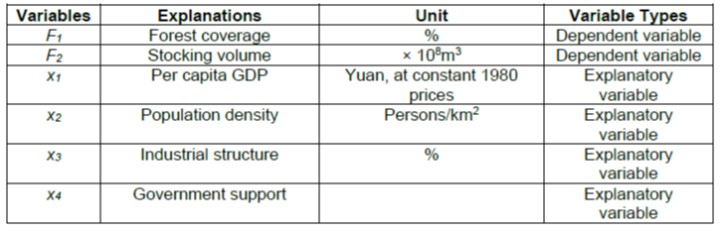Data Preprocessing

Due to the different measurement units and magnitude orders of the determined indicators, errors were likely to occur in the network learning process. Therefore, in order to obtain more accurate modeling results, the data needed to be normalized first, and then the data for all indicators were converted to [0 ,1], according to the conversion Eq. 1:

When “the higher is better” for the indicator attributed value, then: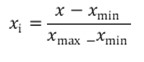(1)

Otherwise: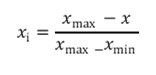(2)

where xi is the standardized data, x is the original data, and xmax and xmin are the maximum and minimum values in the original indicators data, respectively.

Methods

In this study, RBF neural network out of the neural networks was used for the modeling and analysis. The RBF neural network, proposed by Powell in 1985, is a three-layer forward network, mapping from input to output was nonlinear, and mapping from hidden layer space to output space was linear. In addition, RBF belongs to neural network of local approximation. Therefore, identification of RBF neural network on RBF neural network can greatly accelerate network learning speed, and local minimum problems can be locally avoided (Mellit and Benghanem 2007; Ye et al. 2015). The structure of RBF network is shown in Fig. 1.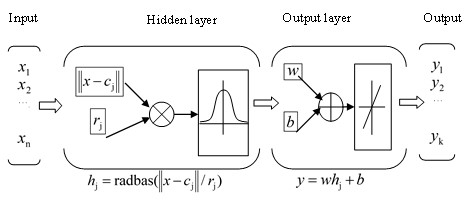Fig. 1. Model of RBF neural network

The first layer is the input layer. Each node of this layer is directly connected with each component of the input vector xi, playing the role of transmitting the input data to the next layer, and the number of nodes is n.

The second layer is the hidden layer. Each node is an RBF node, which represents a single radial basis function related to the center position and expansion constant, and the input data is processed through the radial basis function as the transfer function. The most commonly used radial basis function is the Gaussian function, as shown in Fig. 2. The Euclidean distance between the input vector (x) and the center of the radial basis function is calculated to realize the nonlinear transmission of data in the hidden layer. As shown in Eq. 3,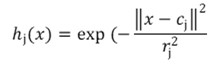(3)

where hj(x) is the output at the jth RBF node, and cj and rj are the center value and width at the jth RBF node respectively.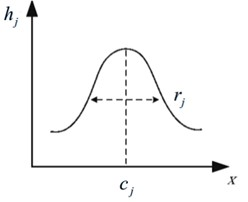Fig. 2. Gaussian basis function

The third layer is the output layer. It is a linear unit which realizes network output, as represented by Eq. 4,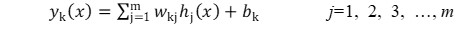(4)

where yk(x) is the kth output of the network to the input vector (x), m is the number of hidden nodes, wkj is the connection weight between the kth output node and the jth hidden node, and bk is the basis.

The algorithmic idea of RBF neural network is as follows: The radial basis function is used for calculating and data transferring units of the hidden unit, and the hidden layer is used to transform the input vector, transforming the low-dimensional mode input data into the high-dimensional space. By this sequence, a problem of linear inseparability in low-dimensional space is transformed into a linear separable problem in high-dimensional space.

RBFNN (RBF Neural Network) Modeling

In this study, the RBFNN model structure of forest resources and social economic factors constructed in MATLAB software (MathWorks, Natick, MA, USA) is shown in Fig. 3.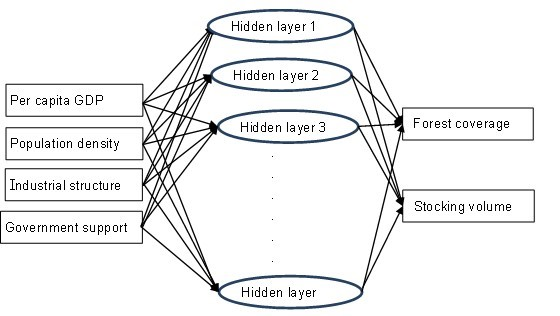Fig. 3. RBFNN model of forest resource and socio-economic factors

By taking the four influencing factors of per capita GDP, population density, industrial structure, and government support as the input vectors of the RBF network training sample, and the forest coverage as well as the stocking volume as the output vector of the RBF network training sample respectively, a network was created to train the training sample data. The training process is shown in Fig. 4.

The data center of the RBF network was obtained by the K-means clustering method; the number of hidden units was determined by the test data criterion: the “best” number of hidden units refers to the number that produces the least error in the test data. Taking 25 groups of data as the training sample set, and the other 14 groups as the validation sample set, thus the ratio between the training set and the validation set was 7:3.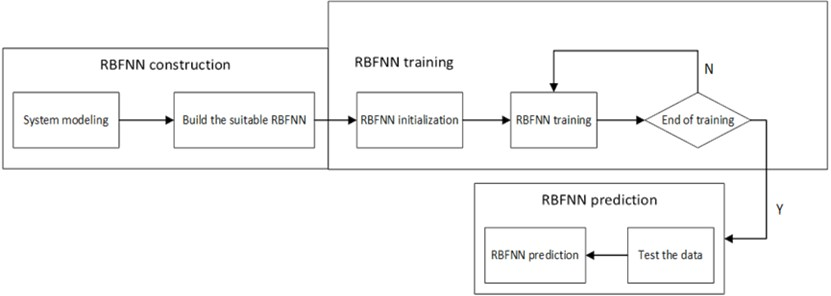Fig. 4. The training process of RBF neural network

Modeling Analysis by Multiple-Linear Regression

In order to verify the superiority of the RBF method in dealing with the nonlinear problems of forest resources, the multiple-linear regression model was built based on the same input data and compared with the modeling results from the RBF neural network. The multiple-linear regression equation constructed in this paper is as follows,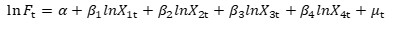(5)

where t is the time index, F denotes the forest coverage or stocking volume, respectively, x1, x2, x3, and x4 represent the real per-capita GDP at the 1980 constant price, popularity density, industry structure, and government support respectively, α indicates the intercept effect, μt is a random error representing all other factors that may influence forest resources, and β1 to β4 are the coefficients to be estimated.

Factor Importance Analysis by Grey Relational Analysis

In order to validate the effectiveness of the RBF method in analyzing the importance of influential factors, the grey relational analysis method has been employed (Deng 1982). One of the advantages of the grey relational analysis is that it can find the major influencing factors of the problem through data processing when only small amounts of data are needed and with no necessity to consider the endogenous problem between variables (Wu and Chen 2005).

The calculation method and steps were as follows: 1) First determine the reference sequence and the comparability sequence. In this paper, the forest coverage and stocking volume were used as a reference sequence respectively, and the other four influencing factors were comparability sequences. 2) Normalize the original data and transform it into a comparable series (this study adopts the mean-value transformation). 3) Calculate the grey relational coefficient between each comparability sequence and the reference sequence, the calculation formula is as follows,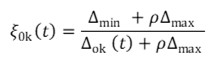(6)

where ∆0k(t) is the deviation sequence of the reference sequence and the comparability sequence.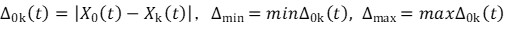(7)

In Eq. 7, X0 denotes the reference sequence, Xk denotes the comparability sequence, and ρ is the identification coefficient: ρ ∈ [0, 1] (the value may be adjusted based on the actual system requirements). A value of ρ is the smaller and the distinguished ability is the larger. Generally, ρ = 0.5 is used.

Finally, according to ξ, the average value of the grey relational coefficient at each time can be obtained, which is the grey relational grade γ0k.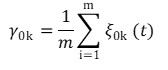(8)

The grey relational grade γ0k represents the level of correlation between the reference sequence and the comparability sequence. If the two sequences are identical, then the value of grey relational grade is equal to 1. The grey relational grade also indicates the degree of influence that the comparability sequence could exert over the reference sequence. Therefore, if a particular comparability sequence is more important than the other comparability sequences to the reference sequence, then the grey relational grade for that comparability sequence and reference sequence will be higher than other grey relational grades (Tosun 2006).

RESULTS AND DISCUSSION

Comparative Analysis of the Results

Tables 2 and 3 and Figs. 5 and 6 show the training performance of the forest coverage and the stocking volume by using both the RBF model and the multiple-linear regression model. Figures 5 and 6 show that the RBF model had a higher degree of fitting to the training samples and can better reflect the trend of the forest coverage and the stocking volume with the years. Tables 2 and 3 show that the RBF model had the small fitting errors for forest coverage and stocking volume, the mean absolute percentage errors (MAPE) were 0.8835% and 1.1137%, respectively, which were much smaller than the 6.8761% and 7.502% of the multiple-linear regression model, showing that RBF has a higher processing capability for input multivariate nonlinear variables.

Table 2. RBF Training and Multiple-linear Regression (Forest Coverage)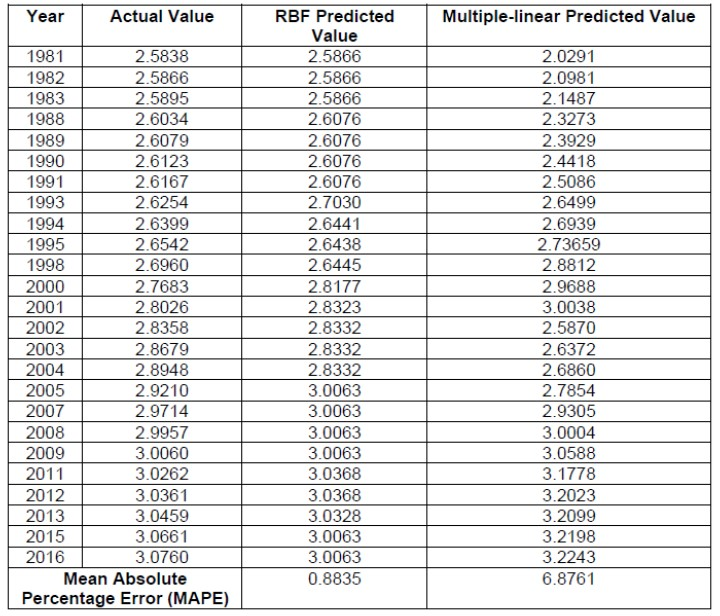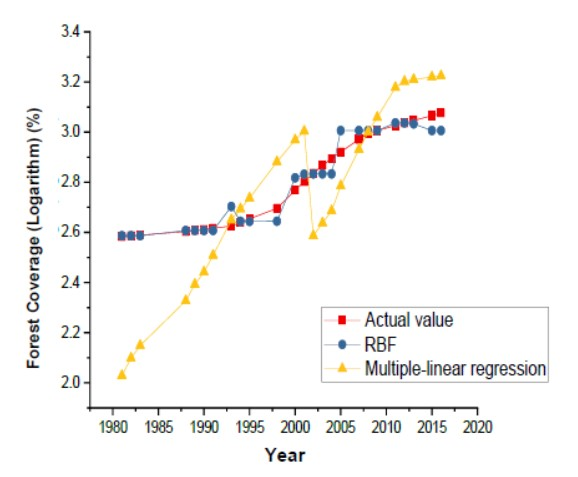Fig. 5. Training results for the forest coverage

Table 3. RBF Training and Multiple-linear Regression (Stocking Volume)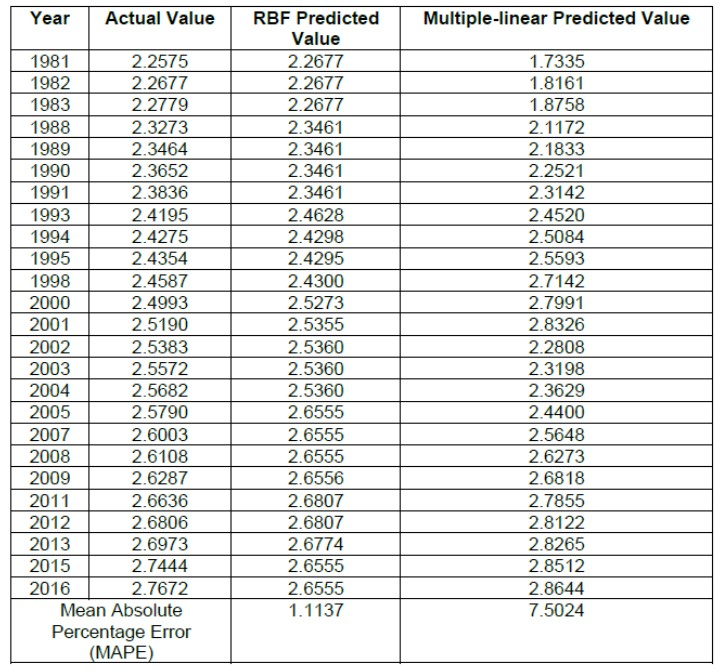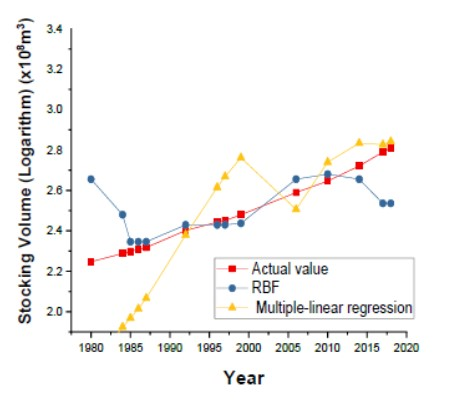Fig. 6. Training results for the stocking volume

The performance of the multiple-linear regression model and the RBF model in the test set is shown in Table 4, Table 5, Fig. 7, and Fig. 8. The RBF model had a higher accuracy and could make better predictions to the changes in forest coverage and stocking volume with the years, with relatively low errors, the mean absolute percentage errors (MAPE) were 3.585% and 4.352%, respectively, which was much lower than the 7.953% and 8.512% from the multiple-linear regression model.

In summary, the accuracy of the RBF model in both the training set and the validation set was better than the multiple-linear regression model, indicating that the RBF model has the advantages in dealing with multivariate-nonlinear modeling and can be used to model the forest resource changes with nonlinear relationship at a high accuracy.

Table 4. Prediction of RBF and Multiple-linear Regression (Forest Coverage)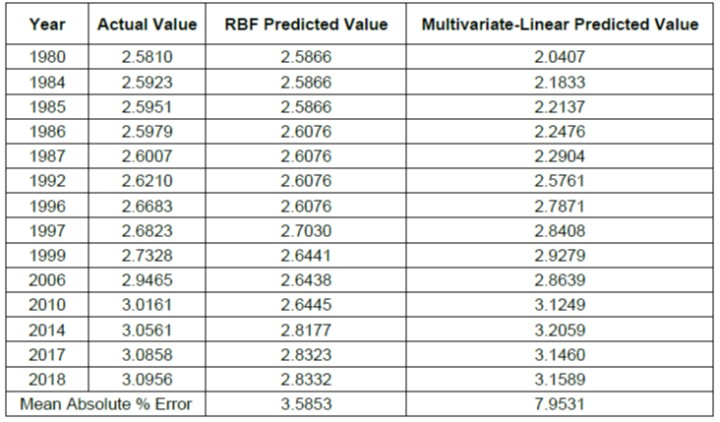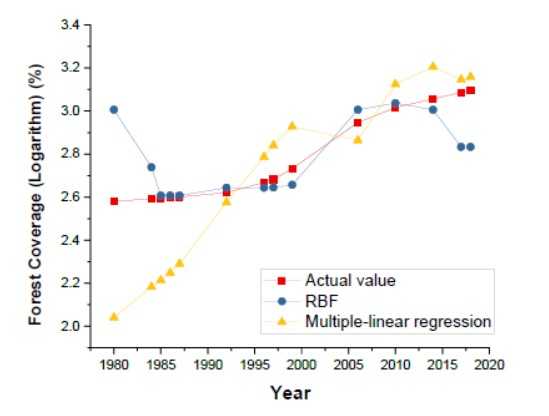Fig. 7. Predicted results of forest coverage

Table 5. Prediction Results of RBF and Multiple-linear Regression (Stocking Volume)